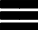Krivalar TutorialsKrivalar Tutorials

# Java if nested inside if statement in programming

## Nested if Statement

Example of Nested if Statement:

``````
public class NestedIf {
public static void main(String a[]){
int sides=4;
int side1,side2,side3,side4;
side1=side2=side3=side4=5;
int angle1,angle2,angle3,angle4;
angle1=angle2=angle3=angle4=90;

if(sides == 4 )
{
System.out.println(
+ "parallelogram,\n rectangle,"
+ " rhombus or square");

if(side1==side2
&& side2==side3
&& side3==side4)
{

System.out.println(
"It can be rhombus or square");

if(angle1==angle2
&& angle2==angle3
&& angle3==angle4)
{
System.out.println(
"It is a square");
}
}
}
}
}``````
This will print
```It can be a quadrilateral, parallelogram,
rectangle, rhombus or square
It can be rhombus or square
It is a square```

## Multiple if-else-if statements

if-else-if statement can be chained to check conditions and take different actions for each condition

``````
public class MultipleIfElseIf {
public static void main(String a[]){
int marks=95;
if(marks<70){
System.out.println("Did not qualify");
}
else if(marks<80){
System.out.println("Average");
}
else if(marks<90){
System.out.println("Good");
}
else{
System.out.println("Excellent");
}
}
}``````

This will print:

`Excellent`

In the above code, if condition is checked and if it returns true, the statement within the block is executed. This is followed by the sequence of else if statements and finally the else statement.